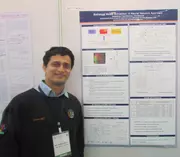X
Voltage and temperature scalable standard cell leakage models based on stacks for statistical leakage characterization
Das B.P., Amrutur B.
Published in IEEE
2008
Pages: 667 - 672
Abstract
With extensive use of Dynamic Voltage Scaling (DVS) there is increasing need for voltage scalable models. Similarly, leakage being very sensitive to temperature motivates the need for a temperature scalable model as well. We characterize standard cell libraries for statistical leakage analysis based on models for transistor stacks. Modeling stacks has the advantage of using a single model across many gates there by reducing the number of models that need to be characterized. Our experiments on 15 different gates show that we needed only 23 models to predict the leakage across 126 input vector combinations. We investigate the use of neural networks for the combined PVT model, for the stacks, which can capture the effect of inter die, intra gate variations, supply voltage(0.6-1.2V) and temperature(0 - 100°C) on leakage. Results show that neural network based stack models can predict the PDF of leakage current across supply voltage and temperature accurately with the average error in mean being less than 2% and that in standard deviation being less than 5% across a range of voltage, temperature. © 2008 IEEE.
•